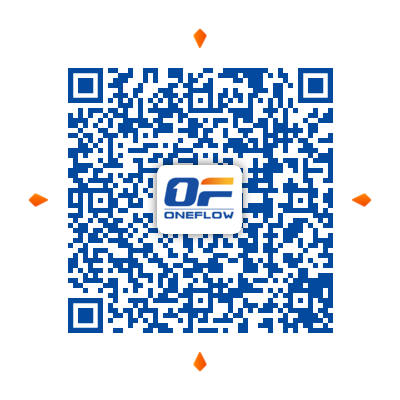# QUICKSTART¶

This section will take the training process of FashionMNIST as an example to briefly show how OneFlow can be used to accomplish common tasks in deep learning. Refer to the links in each section to the presentation on each subtask.

Let’s start by importing the necessary libraries:

import oneflow as flow
import oneflow.nn as nn
from flowvision import transforms
from flowvision import datasets

FlowVision is a tool library matching with OneFlow, specific to computer vision tasks. It contains a number of models, data augmentation methods, data transformation operations and datasets. Here we import and use the data transformation module transforms and datasets module datasets provided by FlowVision.

Settting batch size and device：

BATCH_SIZE=64

DEVICE = "cuda" if flow.cuda.is_available() else "cpu"
print("Using {} device".format(DEVICE))


OneFlow has two primitives to load data, which are Dataset and DataLoader.

The flowvision.datasets module contains a number of real data sets (such as MNIST, CIFAR 10, FashionMNIST).

We can use flowvision.datasets.FashionMNIST to get the training set and test set data of FashionMNIST.

training_data = datasets.FashionMNIST(
root="data",
train=True,
transform=transforms.ToTensor(),
source_url="https://oneflow-public.oss-cn-beijing.aliyuncs.com/datasets/mnist/Fashion-MNIST/",

)
test_data = datasets.FashionMNIST(
root="data",
train=False,
transform=transforms.ToTensor(),
source_url="https://oneflow-public.oss-cn-beijing.aliyuncs.com/datasets/mnist/Fashion-MNIST/",
)


Out:

Downloading https://oneflow-public.oss-cn-beijing.aliyuncs.com/datasets/mnist/Fashion-MNIST/train-images-idx3-ubyte.gz to data/FashionMNIST/raw/train-images-idx3-ubyte.gz
26422272/? [00:15<00:00, 2940814.54it/s]
Extracting data/FashionMNIST/raw/train-images-idx3-ubyte.gz to data/FashionMNIST/raw
...


The data will be downloaded and extracted to./data directory.

The oneflow.utils.data.DataLoader wraps an iterable around the dataset.

train_dataloader = flow.utils.data.DataLoader(
training_data, BATCH_SIZE, shuffle=True
)
test_data, BATCH_SIZE, shuffle=False
)

print("x.shape:", x.shape)
print("y.shape:", y.shape)
break


Out:

x.shape: flow.Size([64, 1, 28, 28])
y.shape: flow.Size()


## Building Networks¶

To define a neural network in OneFlow, we create a class that inherits from nn.Module. We define the layers of the network in the __init__ function and specify how data will pass through the network in the forward function.

class NeuralNetwork(nn.Module):
def __init__(self):
super(NeuralNetwork, self).__init__()
self.flatten = nn.Flatten()
self.linear_relu_stack = nn.Sequential(
nn.Linear(28*28, 512),
nn.ReLU(),
nn.Linear(512, 512),
nn.ReLU(),
nn.Linear(512, 10),
)

def forward(self, x):
x = self.flatten(x)
logits = self.linear_relu_stack(x)
return logits

model = NeuralNetwork().to(DEVICE)
print(model)


Out:

NeuralNetwork(
(flatten): Flatten(start_dim=1, end_dim=-1)
(linear_relu_stack): Sequential(
(0): Linear(in_features=784, out_features=512, bias=True)
(1): ReLU()
(2): Linear(in_features=512, out_features=512, bias=True)
(3): ReLU()
(4): Linear(in_features=512, out_features=10, bias=True)
)
)


## Training Models¶

To train a model, we need a loss function (loss_fn) and an optimizer (optimizer). The loss function is used to evaluate the difference between the prediction of the neural network and the real label. The optimizer adjusts the parameters of the neural network to make the prediction closer to the real label (expected answer). Here, we use oneflow.optim.SGD to be our optimizer. This process is called back propagation.

loss_fn = nn.CrossEntropyLoss().to(DEVICE)
optimizer = flow.optim.SGD(model.parameters(), lr=1e-3)


The train function is defined for training. In a single training loop, the model makes forward propagation, calculates loss, and backpropagates to update the model's parameters.

def train(iter, model, loss_fn, optimizer):
size = len(iter.dataset)
for batch, (x, y) in enumerate(iter):
x = x.to(DEVICE)
y = y.to(DEVICE)

# Compute prediction error
pred = model(x)
loss = loss_fn(pred, y)

# Backpropagation
loss.backward()
optimizer.step()

current = batch * BATCH_SIZE
if batch % 100 == 0:
print(f"loss: {loss:>7f}  [{current:>5d}/{size:>5d}]")


We also define a test function to verify the accuracy of the model:

def test(iter, model, loss_fn):
size = len(iter.dataset)
num_batches = len(iter)
model.eval()
test_loss, correct = 0, 0
for x, y in iter:
x = x.to(DEVICE)
y = y.to(DEVICE)

pred = model(x)
test_loss += loss_fn(pred, y)
bool_value = (pred.argmax(1).to(dtype=flow.int64)==y)
correct += float(bool_value.sum().numpy())
test_loss /= num_batches
print("test_loss", test_loss, "num_batches ", num_batches)
correct /= size
print(f"Test Error: \n Accuracy: {(100*correct):>0.1f}, Avg loss: {test_loss:>8f}")


We use the train function to begin the train process for several epochs and use the test function to assess the accuracy of the network at the end of each epoch:

epochs = 5
for t in range(epochs):
print(f"Epoch {t+1}\n-------------------------------")
print("Done!")


Out:

Epoch 1
-------------------------------
loss: 2.152148  [    0/60000]
loss: 2.140148  [ 6400/60000]
loss: 2.147773  [12800/60000]
loss: 2.088032  [19200/60000]
loss: 2.074728  [25600/60000]
loss: 2.034325  [32000/60000]
loss: 1.994112  [38400/60000]
loss: 1.984397  [44800/60000]
loss: 1.918280  [51200/60000]
loss: 1.884574  [57600/60000]
test_loss tensor(1.9015, device='cuda:0', dtype=oneflow.float32) num_batches  157
Test Error:
Accuracy: 56.3, Avg loss: 1.901461
Epoch 2
-------------------------------
loss: 1.914766  [    0/60000]
loss: 1.817333  [ 6400/60000]
loss: 1.835239  [12800/60000]
...


Use oneflow.save to save the model. The saved model can be then loaded by oneflow.load to make predictions.

flow.save(model.state_dict(), "./model")


## QQ Group¶

Any problems encountered during the installation or usage, welcome to join the QQ Group to discuss with OneFlow developers and enthusiasts:

Add QQ group by 331883 or scan the QR code below: